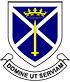# Mathematics

## Department Aims

### At St Albans, regardless of the topic being covered, it is the aim of those teaching Mathematics to provide opportunities for all students to:

• Use and apply mathematics in practical tasks, in real-life problems and within mathematics itself
• Work on problems that pose a challenge
• Encounter and consider different lines of mathematical argument
• Succeed
• Enjoy mathematics

### The Mathematics Department seeks to ensure that each child learns effectively through a number of strategies and teaching styles such as:

• Problem solving
• Class discussion
• Individual and Group work
• Practical work
• Book and worksheets
• Show me boards
• Graphic Calculators

We have taken out a subscription to the website www.mymaths.co.uk. This is a good source of material for use within the lessons but can also be accessed at home by parents and students.

### Years 7 - 11

In Years 7 - 11 the students are taught in sets, but it is our policy to allow students to move sets when appropriate.

Classes will broadly study the modules in this sequence, although there will be variation according to the needs of individual classes, such as Revision in Year 11:

 Autumn Term Graphs and Sequencing Number Proportion Angles Equations then Formulae Construction

 Spring Term Calculations Mensuration Proportion Probability Sequences then Graphs

 Summer Term Term Transformations Data Proportion Equations then Formulae Angles Number

### A Level Mathematics

A Level Mathematics students will study towards the Edexcel (Pearson) specification.
A Level Further Mathematics will study towards the AQA specification.

An outline of the two courses are as follows:

Mathematics
Mathematical argument, language and proof
Mathematical problem solving
Mathematical modelling
Proof
Algebra and functions
Coordinate geometry in the (x,y) plane
Sequences and series
Trigonometry
Exponentials and logarithms
Differentiation
Integration
Numerical methods
Vectors
Statistical sampling
Data presentation and interpretation
Probability
Statistical distributions
Statistical hypothesis testing
Quantities and units in mechanics
Kinematics
Forces and Newton's laws
Moments

This will be assessed by three two-hour examinations at the end of the two-year course.

Further Mathematics
Proof
Complex numbers
Matrices
Further Algebra and Functions
Further Calculus
Further Vectors
Polar coordinates
Hyperbolic functions
Differential equations
Trigonometry
Numerical Methods
Discrete Mathematics
Further Mechanics

This will be assessed by three two-hour examinations at the end of the two-year course.

We offer the one-year level-3 course in Mathematical Studies (sometimes called Core Mathematics). This course is focussed on problem solving and the application of mathematics.  This is ideal for students who are studying A-levels in subjects such as geography, psychology, sociology and history which now have increased mathematical content.